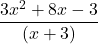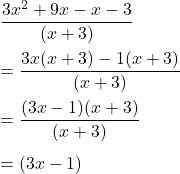## what is the quotient (3x^2 + 8x -3) divided by (x + 3)

Question

what is the quotient (3x^2 + 8x -3) divided by (x + 3)

in progress 0
6 months 2021-08-24T12:57:08+00:00 1 Answers 4 views 0

## Answers ( )

Quotient = (3x-1)

Step-by-step explanation:

we need to find the quotient when (3x² + 8x -3) is divided by (x+3)

It means we need to divide (3x² + 8x -3) by (x+3).

We can write it as follows :Applying middle term splitting on the numerator.So, the required quotient is (3x-1)